• +91 9971497814
• info@interviewmaterial.com

# RD Chapter 30- Derivatives Ex-30.1 Interview Questions Answers

### Related Subjects

Question 1 : Find the derivative of f(x) = 3x at x = 2

Given:
f(x) = 3x
By using the derivative formula,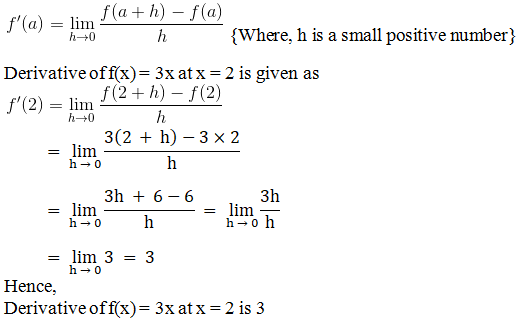Question 2 :

Find the derivativeof f(x) = x2 – 2 at x = 10

Given:

f(x) = x2 – 2

By using the derivative formula,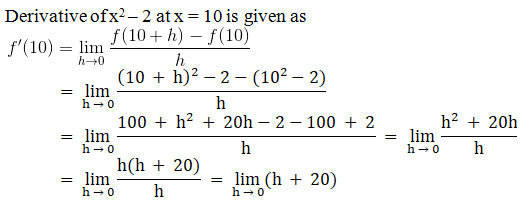= 0 + 20 = 20

Hence,

Derivative of f(x) = x2 –2 at x = 10 is 20

Question 3 : Find the derivative of f(x) = 99x at x = 100.

Given:
f(x) = 99x
By using the derivative formula,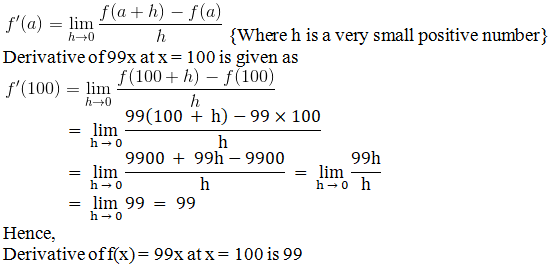Question 4 : Find the derivative of f(x) = x at x = 1

Given:
f(x) = x
By using the derivative formula,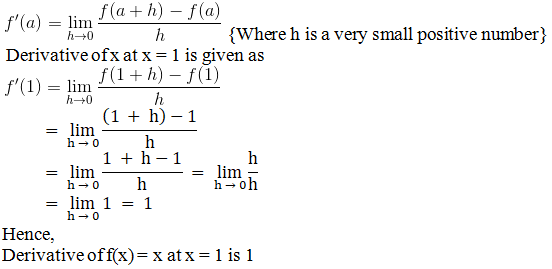Question 5 : Find the derivative of f(x) = cos x at x = 0

Solution:
Given:
f(x) = cos x
By using the derivative formula,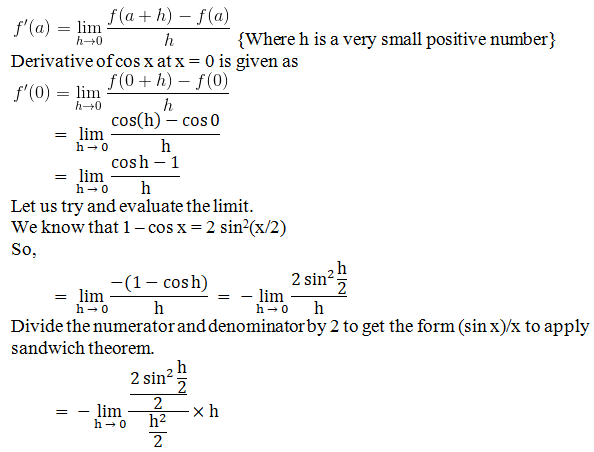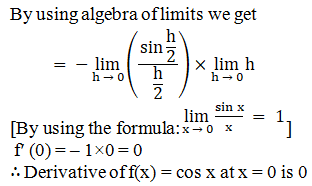Question 6 : Find the derivative of f(x) = tan x at x = 0

Given:
f(x) = tan x
By using the derivative formula,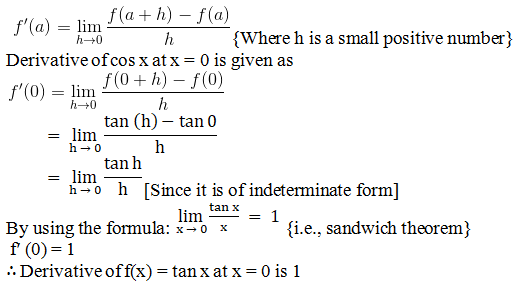Question 7 :
Find the derivatives of the following functions at the indicated points:
(i) sin x at x = π/2
(ii) x at x = 1
(iii) 2 cos x at x = π/2
(iv) sin 2xat x = π/2

(i) sin x at x = π/2
Given:
f (x) = sin x
By using the derivative formula,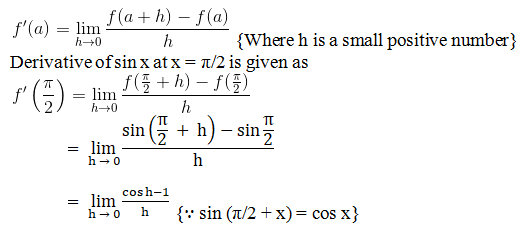[Since it is ofindeterminate form. Let us try to evaluate the limit.]

We know that 1 – cos x = 2 sin2(x/2)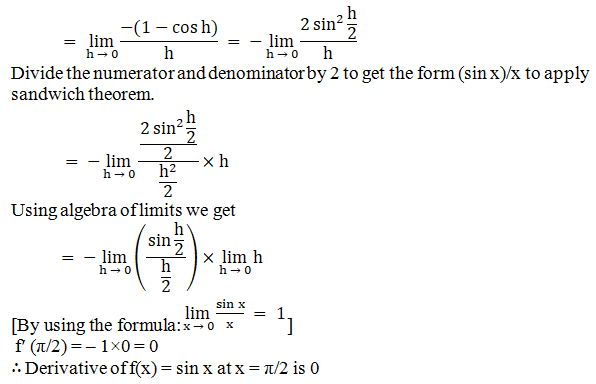(ii) x at x = 1

Given:

f (x) = x

By using the derivative formula,(iii) 2 cos x at x = π/2
Given:
f (x) = 2 cos x
By using the derivative formula,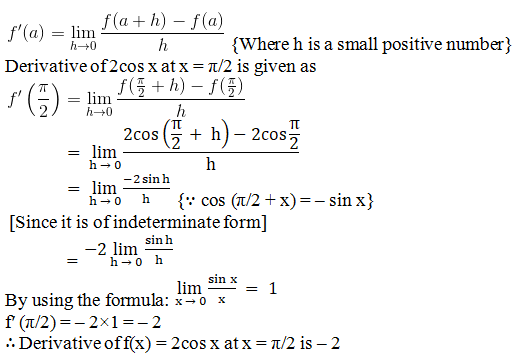(iv) sin 2xat x = π/2
Solution:
Given:
f (x) = sin 2x
By using the derivative formula,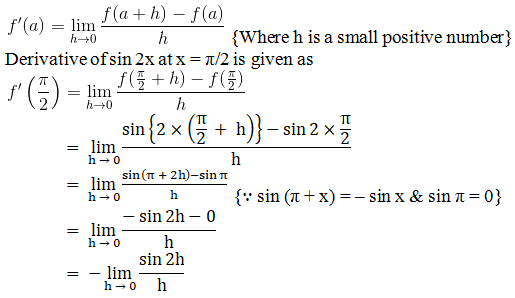[Since it is of indeterminate form. We shall apply sandwich theorem to evaluate the limit.]
Now, multiply numerator and denominator by 2, we get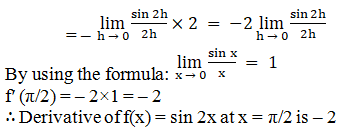Todays Deals### RD Chapter 30- Derivatives Ex-30.1 Contributorskrishan

Name:
Email:

# Latest News# 9000 interview questions in different categories